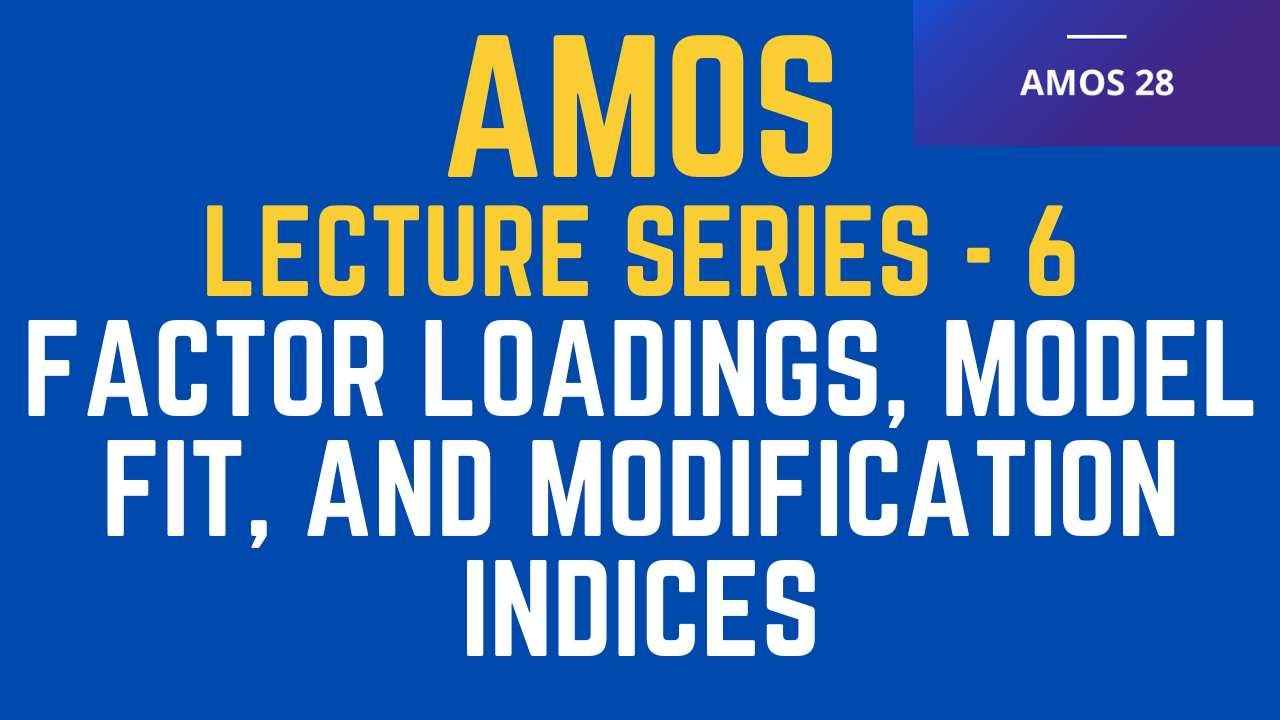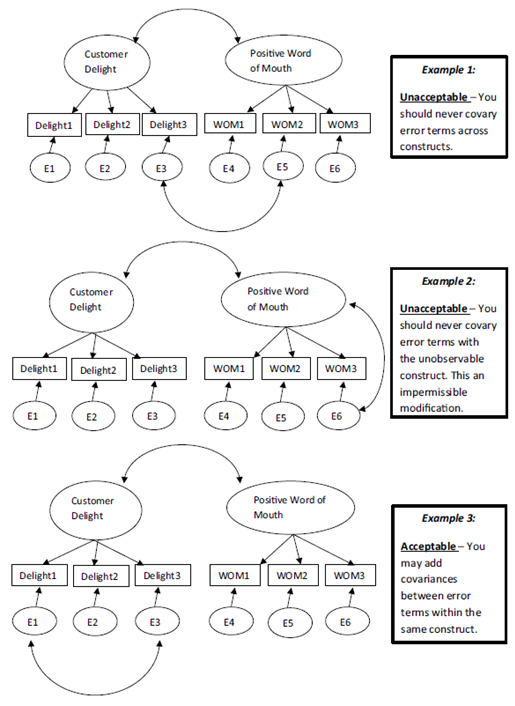## What Will be Discussed?

The tutorial will discuss in detail the concept of factor loadings, acceptable factor loadings, model fit statistics, acceptable model fit statistics to establish a good fit, and how to use Modification Indices to improve model fit.### IBM SPSS AMOS Series - 6

In CB-SEM, Loadings, Model Fit, and Modification Indices are critical to model identification. This post will discuss in detail all these concept.

• The factor loadings in a CFA estimate the direct effects of unobservable constructs on their indicators.
• While unstandardized estimates can be insightful, they are rarely reported in the results of a CFA. Standardized estimates are most frequently reported because they allow you to compare the weights of indicators across a CFA.
• Standardizing an estimate converts your factor loading to a 0 to 1 scale, which allows for an easier comparison of indicators.
• Additionally, squaring the standardized factor loading will give the proportion of explained variance (R2) with each indicator.
• This lets you know how much of the variance in the indicator is explained by the unobserved construct. For instance, if a standardized factor loading is .80, then the unobserved variable explains .802 = .64, or 64% of the variance of the indicator.
• How do I know if I have an acceptable indicator? If you have a standardized factor loading that is greater than .70 or explains at least half of the variance in the indicator (.702 =.50), then your indicator is providing value in explaining the unobserved construct.
• If you are not explaining at least half of the variance, that indicator is contributing little to the understanding of the unobservable construct.
• Once we have determined the standardized value for each factor loading, we can also determine the measurement error for each indicator.
• The measurement error for each indicator is simply 1 − R2. Thus, the lower the explained variance is in an indicator, the higher the measurement error.

• How do I know if I have an acceptable indicator? If you have a standardized factor loading that is greater than .70 or explains at least half of the variance in the indicator (.702 =.50), then your indicator is providing value in explaining the unobserved construct.
• If you are not explaining at least half of the variance, that indicator is contributing little to the understanding of the unobservable construct.
• Once we have determined the standardized value for each factor loading, we can also determine the measurement error for each indicator.
• The measurement error for each indicator is simply 1 − R2. Thus, the lower the explained variance is in an indicator, the higher the measurement error.

## Setting the Metrics

• In SEM, each unobserved variable must be assigned a metric, which is a measurement range.
• This is done by constraining one of the factor loadings from the unobservable variable by assigning it a value of 1.0. The remaining loadings are then free to be estimated.
• The factor loading that is set to 1.0 is acting as a reference point (or range) for the other indicators to be estimated. This process is called “setting the metric”, and the indicator constrained to 1.0 is often referred to as a “reference item”.
• So, which indicator should I constrain to 1.0?
• Researchers normally constrain the first or last indicator of each construct to be set to 1.0.
• If you fail to set the metric or constrain one of your indicators to 1.0, the analysis of your SEM model will not run and will give you an “under-identified” error message.
• Lastly, if you are analyzing and comparing multiple samples, make sure that the same indicator is constrain to 1.0 for each sample.

## Model Fit and Fit Statistics

• One of the advantages of SEM is that you can assess if your model is “fitting” the data or, specifically, the observed covariance matrix.
• The term “model fit” denotes that your specified model (estimated covariance matrix) is a close representation of the data (observed covariance matrix).
• A bad fit, on the other hand, indicates that the data is contrary to the specified model. The model fit test is to understand how the total structure of the model fits the data.
• A good model fit does not mean that every particular part of the model fits well. Again, the test of model fit is looking at the overall model compared to the data.
• One caution with assessing model fit is that a model with fewer indicators per factor will have a higher apparent fit than a model with more indicators per factor. Model fit coefficients reward parsimony.
• Thus, if you have a complex model, you will find it more difficult to achieve a “good model fit” compared to a more simplistic model.
• The AMOS software will give you a plethora of model fit statistics. There are more than 20 different model fit tests, but only the prominent ones seen in most research are discussed.

### Model Fit and Fit Statistics - Chi-Square

• The chi-square test is also called the chi-square goodness of fit test, but in reality, chi-square is a “badness of fit” measure.
• The chi-square value should not be significant if there is a good model fit. A significance means your model’s covariance structure is significantly different from the observed covariance matrix of the data. If chi-square is <.05, then your model is considered to be ill-fitting.
• Chi-square is very sensitive to sample size. In very large samples, even tiny differences between the observed model and the perfect fit model may be found. A better option with chi-square is to use a “relative chi-square” test, which is the chi-square value divided by the degrees of freedom, thus making it less dependent on sample size.
• Kline (2011) states that a relative chi-square test with a value under 3 is considered acceptable fit. Some researchers say that values as high as 5 are okay (Schumacker and Lomax 2004).

### Model Fit and Fit Statistics

• Comparative Fit Index (CFI): CFI varies from 0 to 1. A CFI value close to 1 indicates a good fit. The cutoff for an acceptable fit for a CFI value is > .90 (Bentler and Bonett 1980)—indicating that 90% of the covariation in the data can be reproduced by your model. CFI is not affected by sample size and is a recommend fit statistic to report.
• Incremental Fit Index (IFI): IFI should be .90 or higher to have an acceptable fit. IFI is relatively independent to sample size and is one that is frequently reported.
• Normed Fit Index (NFI): An acceptable fit is above .90 or above. Note that NFI may underestimate fit for small samples and does not reflect parsimony (the more parameters in the model, the larger the NFI).
• Tucker Lewis Index (TLI): This fit index is also called the Non-Normed Fit Index. As with the other fit indices, above .90 equals an acceptable fit.
• Root Mean Square Error of Approximation (RMSEA): This is a “badness of fit” test where values close to “0” equal the best fit. A good model fit is present if RMSEA is below .05. There is an adequate fit if it is .08 and below—values over .10 denote a poor fit (MacCallum et al. 1996).
• Standardized Root Mean Square Residual (SRMR): Like RMSEA, this is a badness of fit test in which the bigger the value, the worse the fit. A SRMR of .05 and below is considered a good fit and a fit of .05 to .09 is considered an adequate fit (MacCallum et al. 1996). This fit statistic has to be specially requested in AMOS compared to other fit statistics that are presented in the analysis. To request this in AMOS, you need to select the “Plugin” tab at the top menu screen and then select “Standard RMR”.

### What Value Means the Model Is a Good Fit?

• There is no shortage of controversy on what should be the cutoff criteria for these fit indices discussed.
• Bentler and Bonett (1980) is the most widely cited research encouraging researchers to pursue model fit statistics (CFI, TLI, NFI, IFI) that are greater than .90.
• This rule of thumb became widely accepted even though researchers such as Hu and Bentler (1999) argued that a .90 criteria was too liberal and that fit indices needed to be greater than .95 to be considered a good-fitting model.
• Subsequently, Marsh et al. (2004) have argued against the rigorous Hu and Bentler criteria in favor of using multiple indices based on the sample size, estimators or distributions.
• Hence, there are no golden rules that universally hold as it pertains to model fit. The criteria outlined in this section is based on the existing literature and provides guidance on what is an “acceptable” model fit to the data.
• Even if a researcher exceeds the .90 threshold for a model fit index, one should use caution in stating a model is a “good” fit. Kline (2011) notes that even if a model is deemed to have a passable model fit, it does not mean that it is correctly specified. A so-called “good-fitting” model can still poorly explain the relationships in a model.

### Modification Indices

• Modification indices are part of the analysis that suggest model alterations to achieve a better fit to the data.
• Making changes via modification indices should be done very carefully and have justification.
• In AMOS, modification indices are concerned with adding additional covariances within a construct’s indicators or relationship paths between constructs. Note that the modification indices option in AMOS will not run if you have missing data.
• In the output of the modification indices, AMOS will list potential changes by adding covariances between error terms and also presenting possible relationships between constructs (listed as regression weights). In a CFA, we are concerned only with covariances between error terms. All other modification indices for a CFA are inappropriate.
• The modification indices will have an initial column that simply says “MI”, which stands for modification indices threshold. This value presented under the MI heading is the reduction of the chi-square value by adding an additional covariance.
• A modification indices threshold value needs to be at least be 3.84 to show a significant difference.
• In AMOS, the default threshold is a value of 4 where any potential modification below this value is not presented.
• As stated, with a CFA, you are concerned only with modification indices related to the covariances, but to clarify this, covariances of indicators within a construct. It is inappropriate to covary indicators across constructs even though the modification indices will suggest it. Below are some suggestions of “dos and don’ts” with modification indices.Source: Collier, J. E. (2020). Applied structural equation modeling using AMOS: Basic to advanced techniques. Routledge.

### References

• Collier, J. E. (2020). Applied structural equation modeling using AMOS: Basic to advanced techniques. Routledge.
• Awang, Z. (2015). A Handbook on SEM (2nd Edition). Malaysia